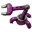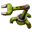## Catalysts should automatically be reused

Post your ideas and suggestions how to improve the game.
Adamo
Filter InserterPosts: 297
Joined: Sat May 24, 2014 7:00 am
Contact:

### Catalysts should automatically be reused

##### TL;DR
There is a "catalyst_amount" value for recipe ingredients/results. In the case where catalysts have some probability of being a result, the assembling-machine entity should automatically re-use the resulting catalyst, if the machine has room.

##### What ?
I'm not entirely clear how the catalyst_amount value in the recipe ingredient and result prototype is used by the engine. I don't see it being used in any base mod prototypes. However, it is there! It can be set on items on both the ingredients and results side of the recipe prototype. One way to use this would be to say that a recipe takes iron and copper plates as catalysts, with some probability that the iron and copper plate survives the recipe as a result. For example,

Code: Select all

``````	ingredients = {{
type="fluid",
name="steam",
amount=100,
--fluidbox_index = 1
},{
type="fluid",
name="adamo-carbon-methane",
amount=100,
--fluidbox_index = 2
},{
type="item",
name="iron-plate",
amount=1,
catalyst_amount = 1
},{
type="item",
name="copper-plate",
amount=1,
catalyst_amount = 1
}},
results = {{
type="fluid",
name="heavy-oil",
amount=1,
--fluidbox_index = 1
},{
type="fluid",
name="light-oil",
amount=5,
--fluidbox_index = 2
},{
type="fluid",
name="petroleum-gas",
amount=20,
--fluidbox_index = 3
},{
type="item",
name="iron-plate",
amount=1,
catalyst_amount = 1,
probability = 0.8
},{
type="item",
name="copper-plate",
amount=1,
catalyst_amount = 1,
probability = 0.6
}}
``````
This is very convenient in the case of a recipe that requires a metal catalyst, but where using an entire plate would be overly expensive in terms of recipe balance. In the case where the recipe does return the catalyst, I recommend that the resulting catalyst be returned to the input chest on the machine producing that recipe. Without that feature, the following setup is necessary:catalyst.png (911.63 KiB) Viewed 80 times
##### Why ?
You can see from my picture that handling this situation is doable using logistics, but in the case where the catalyst_amount is used in both the ingredient and result side of the recipe, I believe it makes sense that the desired effect is to have the catalyst returned to the input. Then, the assembling-machine will only take in new items when the current catalyst items have depleted. Therefore, I recommend this be added to the base game mechanics. Thank you.

Deadlock989
Smart InserterPosts: 1090
Joined: Fri Nov 06, 2015 7:41 pm
Contact:

+1.

### Who is online

Users browsing this forum: No registered users